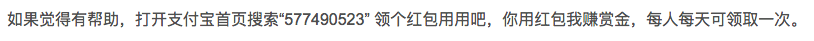# js如何判断一个对象{}是否为空对象，没有任何属性

js如何判断一个对象{}是否为空对象，没有任何属性

if (typeof model.rows === "object" && !(model.rows instanceof Array)){ model.rows = [model.rows];}

model.rows = {};

### 解决方案：

if (typeof model.rows === "object" && !(model.rows instanceof Array)){ var hasProp = false; for (var prop in model.rows){  hasProp = true;  break; } if (hasProp){  model.rows = [model.rows]; }else{  throw "model.rows is empty object";  return false; }}

### jQuery中的实现方法

JavaScript判断object/json 是否为空，可以使用jQuery的isEmptyObject()方法。

function isEmptyObject(e) { var t; for (t in e)  return !1; return !0}

console.log(\$.isEmptyObject({"re": 2}));  //false console.log(isEmptyObject());    //trueconsole.log(isEmptyObject({}));   //trueconsole.log(isEmptyObject(null));  //trueconsole.log(isEmptyObject(23));   //trueconsole.log(isEmptyObject({"te": 2}));  //false

### 结束语

jQuery的isEmptyObject()方法实现的代码即简单又简洁，但最关键的是我们要理解原理。点击重新获取扫码支付余额充值